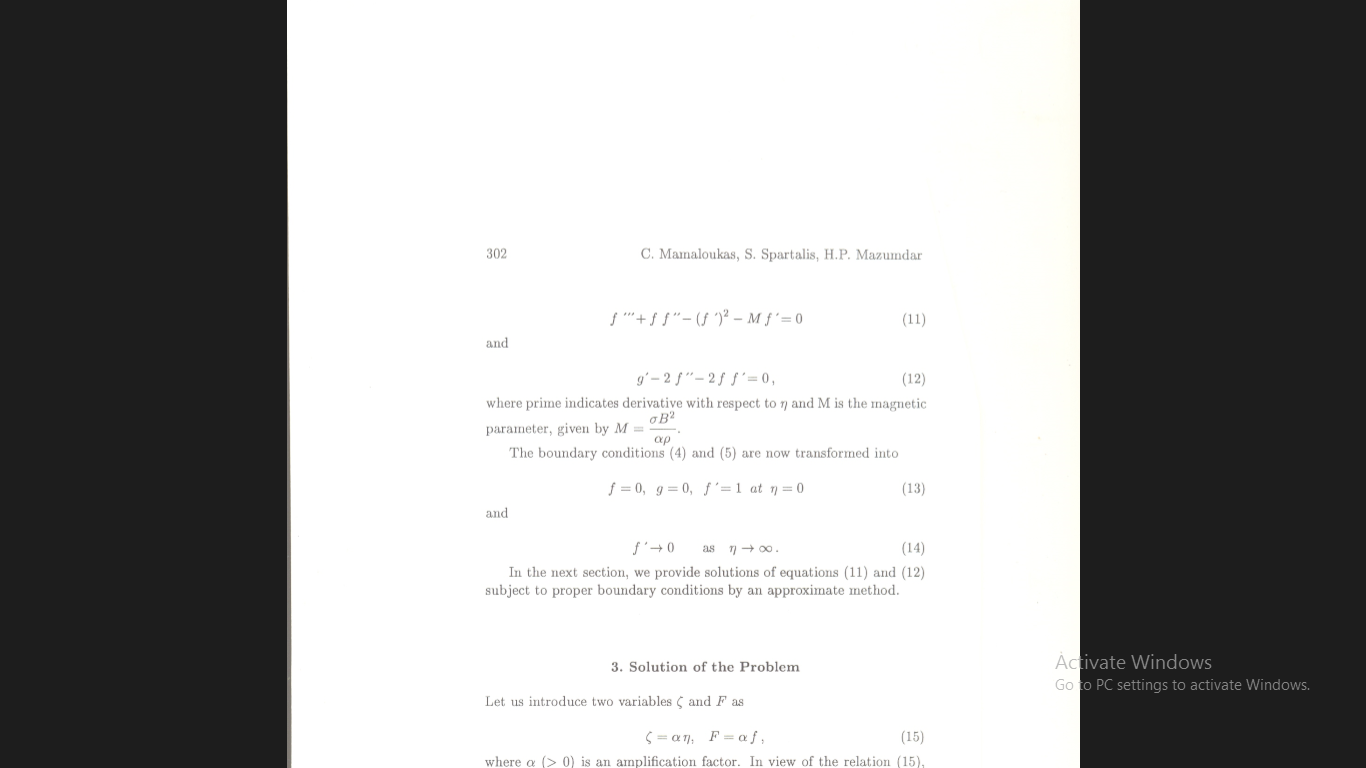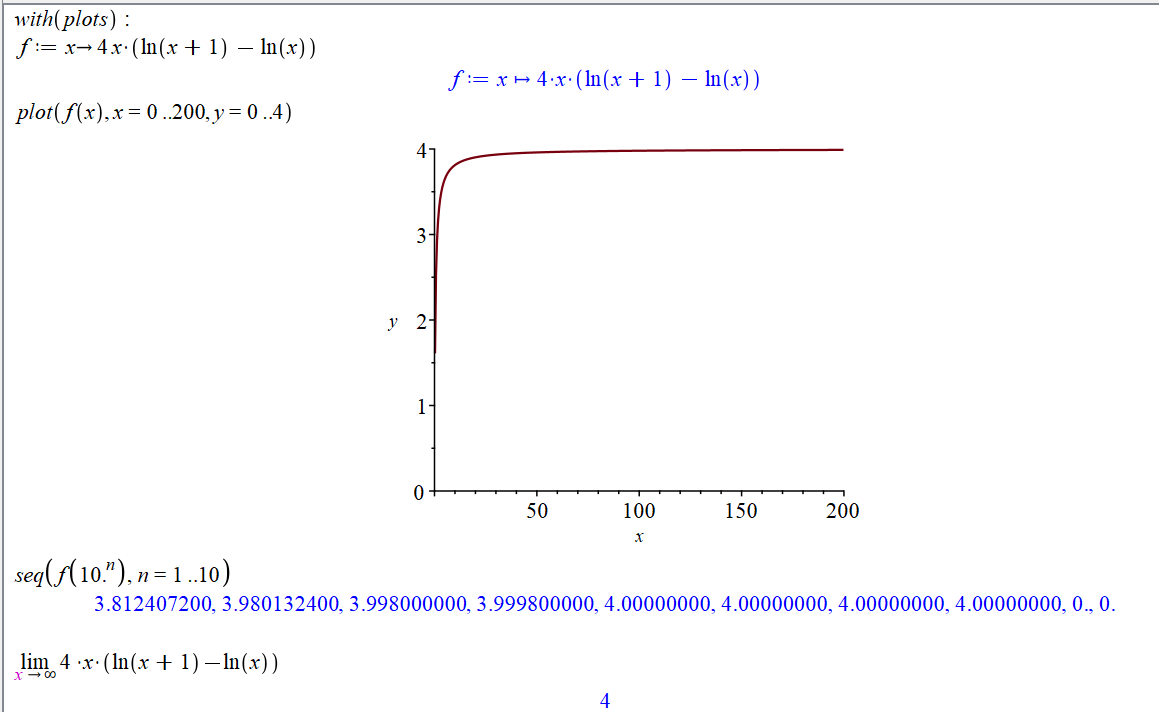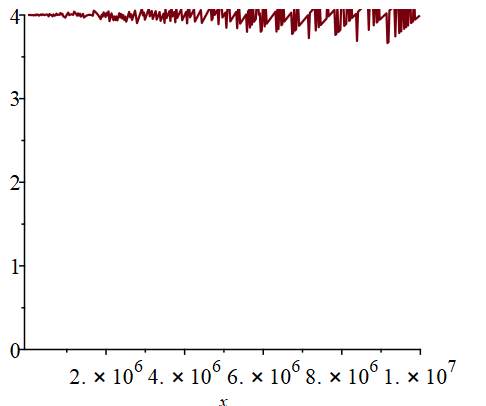<

## How do i calculate this in maple. ...

Have to draw the graph## Can't restore default :-diff after using Physics:-...

What's going on in the following? Why can't I restore the default behavior of diff after using Physics:-diff or even just using with(Physics)? Is it because of Physics:-ModuleLoad()?

 > restart:
 > diff(conjugate(f(x)), x);> Physics:-diff(conjugate(f(x)), x);> forget(.., conjugate(f(x)));
 > diff(conjugate(f(x)), x);> restart:
 > with(Physics):
 > :-diff(:-conjugate(f(x)), x);>

I don't know why the prettyprinted output of my worksheet is shown the way that it is above. I didn't do anything differently than I usually do to upload a worksheet. Anyway, the output is simple enough that I think that my Question is still clear.

## Why the integration can not work out when changing...

Dear all,

The program is as follows (The "mw" files are also attached). The integraion "evalf(Int(k*sin(x)*T1,x=0..Pi/2,y=-Pi/6..-Pi/6+afa))" can not be worked out in several hours, but if the upper limit of x is changed to 0.5 (for example), the integration can be worked out quickly. I have tried to change the program to math model, however, the question still exists. How to solve this problem?

afa:=0.3:
vh:=3.5:
u:=3.12:
mu:=5.5:
gama:=-4*10^(-29)*(1-cos(6*afa))*(1-1*10^(-8)*I):
d1:=1.78*10^(-9):
d2:=48.22*10^(-9):
HBAR:=1.05457266*10^(-34):
ME:=9.1093897*10^(-31):
ELEC:=1.60217733*10^(-19):
Kh:=2.95*10^10:
kc:=sqrt(2*ME*ELEC/HBAR^2):
k:=kc*sqrt(mu):
k0:=sqrt(k^2-k^2*sin(x)^2):
kh:=sqrt(k^2-(Kh-k*sin(x)*cos(y))^2 - k^2 * sin(x)^2 * sin(y)^2):
khg:=sqrt(k^2-(2*Kh*sin(afa/2)*sin(afa/2)-k*sin(x)*cos(y))^2-(2*Kh*sin(afa/2)*cos(afa/2)+k*sin(x)*sin(y))^2):
kg1:=sqrt(k^2-(Kh*cos(Pi/3-afa)-k*sin(x)*cos(y))^2-(Kh*sin(Pi/3-afa)+k*sin(x)*sin(y))^2):
kg2:=sqrt(k^2-(Kh*cos(afa)-k*sin(x)*cos(y))^2-(k*sin(x)*sin(y)-Kh*sin(afa))^2):
k0pl:=sqrt(k^2-k^2*sin(x)^2+kc^2*vh-kc^2*u):
k0mi:=sqrt(k^2-k^2*sin(x)^2-kc^2*vh-kc^2*u):
khpl:=sqrt(k^2-(Kh-k*sin(x)*cos(y))^2-k^2*sin(x)^2*sin(y)^2+kc^2*vh-kc^2*u):
khmi:=sqrt(k^2-(Kh-k*sin(x)*cos(y))^2-k^2*sin(x)^2*sin(y)^2-kc^2*vh-kc^2*u):
k0plpl:=sqrt(k^2-k^2*sin(x)^2+2*kc^2*vh):
k0mimi:=sqrt(k^2-k^2*sin(x)^2-2*kc^2*vh):
khplpl:=sqrt(k^2-(Kh-k*sin(x)*cos(y))^2-k^2*sin(x)^2*sin(y)^2+2*kc^2*vh):
khmimi:=sqrt(k^2-(Kh-k*sin(x)*cos(y))^2-k^2*sin(x)^2*sin(y)^2-2*kc^2*vh):
khgplpl:=sqrt(k^2-(2*Kh*sin(afa/2)*sin(afa/2)-k*sin(x)*cos(y))^2-(2*Kh*sin(afa/2)*cos(afa/2)+k*sin(x)*sin(y))^2+2*kc^2*vh):
khgmimi:=sqrt(k^2-(2*Kh*sin(afa/2)*sin(afa/2)-k*sin(x)*cos(y))^2-(2*Kh*sin(afa/2)*cos(afa/2)+k*sin(x)*sin(y))^2-2*kc^2*vh):
kg1plpl:=sqrt(k^2-(Kh*cos(Pi/3-afa)-k*sin(x)*cos(y))^2-(Kh*sin(Pi/3-afa)+k*sin(x)*sin(y))^2+2*kc^2*vh):
kg1mimi:=sqrt(k^2-(Kh*cos(Pi/3-afa)-k*sin(x)*cos(y))^2-(Kh*sin(Pi/3-afa)+k*sin(x)*sin(y))^2-2*kc^2*vh):
kg2plpl:=sqrt(k^2-(Kh*cos(afa)-k*sin(x)*cos(y))^2-(k*sin(x)*sin(y)-Kh*sin(afa))^2+2*kc^2*vh):
kg2mimi:=sqrt(k^2-(Kh*cos(afa)-k*sin(x)*cos(y))^2-(k*sin(x)*sin(y)-Kh*sin(afa))^2-2*kc^2*vh):
A1:=1/(1+I*ME*gama/(HBAR^2*k0pl))*exp(I*k0pl*d1)/2:
B1:=1/(1+I*ME*gama/(HBAR^2*k0pl))*exp(I*k0pl*d1)/2:
A2:=1/(1+I*ME*gama/(HBAR^2*khpl))*exp(I*khpl*d1)/2:
B2:=1/(1+I*ME*gama/(HBAR^2*khpl))*exp(I*khpl*d1)/2:
A3:=1/(1+I*ME*gama/(HBAR^2*k0mi))*exp(I*k0mi*d1)/2:
B3:=1/(1+I*ME*gama/(HBAR^2*k0mi))*exp(I*k0mi*d1)/2:
A4:=1/(1+I*ME*gama/(HBAR^2*khmi))*exp(I*khmi*d1)/2:
B4:=1/(1+I*ME*gama/(HBAR^2*khmi))*exp(I*khmi*d1)/2:
T1:=1/4*Re(abs(A1)^2*k0plpl*exp(I*(k0plpl-conjugate(k0plpl))*d2)+abs(A1)^2*kg1plpl*exp(I*(kg1plpl-conjugate(kg1plpl))*d2)+abs(A2)^2*khplpl*exp(I*(khplpl-conjugate(khplpl))*d2)+abs(A2)^2*khgplpl*exp(I*(khgplpl-conjugate(khgplpl))*d2)+abs(B3)^2*k0mimi*exp(I*(k0mimi-conjugate(k0mimi))*d2)+abs(B3)^2*kg1mimi*exp(I*(kg1mimi-conjugate(kg1mimi))*d2)+abs(B4)^2*khmimi*exp(I*(khmimi-conjugate(khmimi))*d2)+abs(B4)^2*khgmimi*exp(I*(khgmimi-conjugate(khgmimi))*d2)+abs(B1+A3)^2*k0*exp(I*(k0-conjugate(k0))*d2)+abs(B1-A3)^2*kg1*exp(I*(kg1-conjugate(kg1))*d2)+abs(A4-B2)^2*kh*exp(I*(kh-conjugate(kh))*d2)+abs(A4+B2)^2*khg*exp(I*(khg-conjugate(khg))*d2)+conjugate(A1)*B3*k0mimi*exp(I*(k0mimi-conjugate(k0plpl))*d2)+A1*conjugate(B3)*k0plpl*exp(I*(k0plpl-conjugate(k0mimi))*d2)+conjugate(A1)*(B1+A3)*k0*exp(I*(k0-conjugate(k0plpl))*d2)+A1*conjugate(B1+A3)*k0plpl*exp(I*(k0plpl-conjugate(k0))*d2)+conjugate(B3)*(B1+A3)*k0*exp(I*(k0-conjugate(k0mimi))*d2)+B3*conjugate(B1+A3)*k0mimi*exp(I*(k0mimi-conjugate(k0))*d2)-conjugate(A1)*B3*kg1mimi*exp(I*(kg1mimi-conjugate(kg1plpl))*d2)-A1*conjugate(B3)*kg1plpl*exp(I*(kg1plpl-conjugate(kg1mimi))*d2)+conjugate(A1)*(A3-B1)*kg1*exp(I*(kg1-conjugate(kg1plpl))*d2)+A1*conjugate(A3-B1)*kg1plpl*exp(I*(kg1plpl-conjugate(kg1))*d2)+conjugate(B3)*(B1-A3)*kg1*exp(I*(kg1-conjugate(kg1mimi))*d2)+B3*conjugate(B1-A3)*kg1mimi*exp(I*(kg1mimi-conjugate(kg1))*d2)-conjugate(A2)*B4*khmimi*exp(I*(khmimi-conjugate(khplpl))*d2)-A2*conjugate(B4)*khplpl*exp(I*(khplpl-conjugate(khmimi))*d2)+conjugate(A2)*(B2-A4)*kh*exp(I*(kh-conjugate(khplpl))*d2)+A2*conjugate(B2-A4)*khplpl*exp(I*(khplpl-conjugate(kh))*d2)+conjugate(B4)*(A4-B2)*kh*exp(I*(kh-conjugate(khmimi))*d2)+B4*conjugate(A4-B2)*khmimi*exp(I*(khmimi-conjugate(kh))*d2)+conjugate(A2)*B4*khgmimi*exp(I*(khgmimi-conjugate(khgplpl))*d2)+A2*conjugate(B4)*khgplpl*exp(I*(khgplpl-conjugate(khgmimi))*d2)-conjugate(A2)*(A4+B2)*khg*exp(I*(khg-conjugate(khgplpl))*d2)-A2*conjugate(A4+B2)*khgplpl*exp(I*(khgplpl-conjugate(khg))*d2)-conjugate(B4)*(A4+B2)*khg*exp(I*(khg-conjugate(khgmimi))*d2)-B4*conjugate(A4+B2)*khgmimi*exp(I*(khgmimi-conjugate(khg))*d2)):
T2:=1/4*Re(abs(A1)^2*k0plpl*exp(I*(k0plpl-conjugate(k0plpl))*d2)+abs(A1)^2*kg2plpl*exp(I*(kg2plpl-conjugate(kg2plpl))*d2)+abs(A2)^2*khplpl*exp(I*(khplpl-conjugate(khplpl))*d2)+abs(A2)^2*khgplpl*exp(I*(khgplpl-conjugate(khgplpl))*d2)+abs(B3)^2*k0mimi*exp(I*(k0mimi-conjugate(k0mimi))*d2)+abs(B3)^2*kg2mimi*exp(I*(kg2mimi-conjugate(kg2mimi))*d2)+abs(B4)^2*khmimi*exp(I*(khmimi-conjugate(khmimi))*d2)+abs(B4)^2*khgmimi*exp(I*(khgmimi-conjugate(khgmimi))*d2)+abs(B1+A3)^2*k0*exp(I*(k0-conjugate(k0))*d2)+abs(B1-A3)^2*kg2*exp(I*(kg2-conjugate(kg2))*d2)+abs(A4-B2)^2*kh*exp(I*(kh-conjugate(kh))*d2)+abs(A4+B2)^2*khg*exp(I*(khg-conjugate(khg))*d2)+conjugate(A1)*B3*k0mimi*exp(I*(k0mimi-conjugate(k0plpl))*d2)+A1*conjugate(B3)*k0plpl*exp(I*(k0plpl-conjugate(k0mimi))*d2)+conjugate(A1)*(B1+A3)*k0*exp(I*(k0-conjugate(k0plpl))*d2)+A1*conjugate(B1+A3)*k0plpl*exp(I*(k0plpl-conjugate(k0))*d2)+conjugate(B3)*(B1+A3)*k0*exp(I*(k0-conjugate(k0mimi))*d2)+B3*conjugate(B1+A3)*k0mimi*exp(I*(k0mimi-conjugate(k0))*d2)-conjugate(A1)*B3*kg2mimi*exp(I*(kg2mimi-conjugate(kg2plpl))*d2)-A1*conjugate(B3)*kg2plpl*exp(I*(kg2plpl-conjugate(kg2mimi))*d2)+conjugate(A1)*(A3-B1)*kg2*exp(I*(kg2-conjugate(kg2plpl))*d2)+A1*conjugate(A3-B1)*kg2plpl*exp(I*(kg2plpl-conjugate(kg2))*d2)+conjugate(B3)*(B1-A3)*kg2*exp(I*(kg2-conjugate(kg2mimi))*d2)+B3*conjugate(B1-A3)*kg2mimi*exp(I*(kg2mimi-conjugate(kg2))*d2)-conjugate(A2)*B4*khmimi*exp(I*(khmimi-conjugate(khplpl))*d2)-A2*conjugate(B4)*khplpl*exp(I*(khplpl-conjugate(khmimi))*d2)+conjugate(A2)*(B2-A4)*kh*exp(I*(kh-conjugate(khplpl))*d2)+A2*conjugate(B2-A4)*khplpl*exp(I*(khplpl-conjugate(kh))*d2)+conjugate(B4)*(A4-B2)*kh*exp(I*(kh-conjugate(khmimi))*d2)+B4*conjugate(A4-B2)*khmimi*exp(I*(khmimi-conjugate(kh))*d2)+conjugate(A2)*B4*khgmimi*exp(I*(khgmimi-conjugate(khgplpl))*d2)+A2*conjugate(B4)*khgplpl*exp(I*(khgplpl-conjugate(khgmimi))*d2)-conjugate(A2)*(A4+B2)*khg*exp(I*(khg-conjugate(khgplpl))*d2)-A2*conjugate(A4+B2)*khgplpl*exp(I*(khgplpl-conjugate(khg))*d2)-conjugate(B4)*(A4+B2)*khg*exp(I*(khg-conjugate(khgmimi))*d2)-B4*conjugate(A4+B2)*khgmimi*exp(I*(khgmimi-conjugate(khg))*d2)):
evalf(Int(k*sin(x)*T1,x=0..Pi/2,y=-Pi/6..-Pi/6+afa))

 > afa:=0.3: vh:=3.5: u:=3.12: mu:=5.5: gama:=-4*10^(-29)*(1-cos(6*afa))*(1-1*10^(-8)*I): d1:=1.78*10^(-9): d2:=48.22*10^(-9): HBAR:=1.05457266*10^(-34): ME:=9.1093897*10^(-31): ELEC:=1.60217733*10^(-19): Kh:=2.95*10^10: kc:=sqrt(2*ME*ELEC/HBAR^2): k:=kc*sqrt(mu): k0:=sqrt(k^2-k^2*sin(x)^2): kh:=sqrt(k^2-(Kh-k*sin(x)*cos(y))^2 - k^2 * sin(x)^2 * sin(y)^2): khg:=sqrt(k^2-(2*Kh*sin(afa/2)*sin(afa/2)-k*sin(x)*cos(y))^2-(2*Kh*sin(afa/2)*cos(afa/2)+k*sin(x)*sin(y))^2): kg1:=sqrt(k^2-(Kh*cos(Pi/3-afa)-k*sin(x)*cos(y))^2-(Kh*sin(Pi/3-afa)+k*sin(x)*sin(y))^2): kg2:=sqrt(k^2-(Kh*cos(afa)-k*sin(x)*cos(y))^2-(k*sin(x)*sin(y)-Kh*sin(afa))^2): k0pl:=sqrt(k^2-k^2*sin(x)^2+kc^2*vh-kc^2*u): k0mi:=sqrt(k^2-k^2*sin(x)^2-kc^2*vh-kc^2*u): khpl:=sqrt(k^2-(Kh-k*sin(x)*cos(y))^2-k^2*sin(x)^2*sin(y)^2+kc^2*vh-kc^2*u): khmi:=sqrt(k^2-(Kh-k*sin(x)*cos(y))^2-k^2*sin(x)^2*sin(y)^2-kc^2*vh-kc^2*u): k0plpl:=sqrt(k^2-k^2*sin(x)^2+2*kc^2*vh): k0mimi:=sqrt(k^2-k^2*sin(x)^2-2*kc^2*vh): khplpl:=sqrt(k^2-(Kh-k*sin(x)*cos(y))^2-k^2*sin(x)^2*sin(y)^2+2*kc^2*vh): khmimi:=sqrt(k^2-(Kh-k*sin(x)*cos(y))^2-k^2*sin(x)^2*sin(y)^2-2*kc^2*vh): khgplpl:=sqrt(k^2-(2*Kh*sin(afa/2)*sin(afa/2)-k*sin(x)*cos(y))^2-(2*Kh*sin(afa/2)*cos(afa/2)+k*sin(x)*sin(y))^2+2*kc^2*vh): khgmimi:=sqrt(k^2-(2*Kh*sin(afa/2)*sin(afa/2)-k*sin(x)*cos(y))^2-(2*Kh*sin(afa/2)*cos(afa/2)+k*sin(x)*sin(y))^2-2*kc^2*vh): kg1plpl:=sqrt(k^2-(Kh*cos(Pi/3-afa)-k*sin(x)*cos(y))^2-(Kh*sin(Pi/3-afa)+k*sin(x)*sin(y))^2+2*kc^2*vh): kg1mimi:=sqrt(k^2-(Kh*cos(Pi/3-afa)-k*sin(x)*cos(y))^2-(Kh*sin(Pi/3-afa)+k*sin(x)*sin(y))^2-2*kc^2*vh): kg2plpl:=sqrt(k^2-(Kh*cos(afa)-k*sin(x)*cos(y))^2-(k*sin(x)*sin(y)-Kh*sin(afa))^2+2*kc^2*vh): kg2mimi:=sqrt(k^2-(Kh*cos(afa)-k*sin(x)*cos(y))^2-(k*sin(x)*sin(y)-Kh*sin(afa))^2-2*kc^2*vh): A1:=1/(1+I*ME*gama/(HBAR^2*k0pl))*exp(I*k0pl*d1)/2: B1:=1/(1+I*ME*gama/(HBAR^2*k0pl))*exp(I*k0pl*d1)/2: A2:=1/(1+I*ME*gama/(HBAR^2*khpl))*exp(I*khpl*d1)/2: B2:=1/(1+I*ME*gama/(HBAR^2*khpl))*exp(I*khpl*d1)/2: A3:=1/(1+I*ME*gama/(HBAR^2*k0mi))*exp(I*k0mi*d1)/2: B3:=1/(1+I*ME*gama/(HBAR^2*k0mi))*exp(I*k0mi*d1)/2: A4:=1/(1+I*ME*gama/(HBAR^2*khmi))*exp(I*khmi*d1)/2: B4:=1/(1+I*ME*gama/(HBAR^2*khmi))*exp(I*khmi*d1)/2: T1:=1/4*Re(abs(A1)^2*k0plpl*exp(I*(k0plpl-conjugate(k0plpl))*d2)+abs(A1)^2*kg1plpl*exp(I*(kg1plpl-conjugate(kg1plpl))*d2)+abs(A2)^2*khplpl*exp(I*(khplpl-conjugate(khplpl))*d2)+abs(A2)^2*khgplpl*exp(I*(khgplpl-conjugate(khgplpl))*d2)+abs(B3)^2*k0mimi*exp(I*(k0mimi-conjugate(k0mimi))*d2)+abs(B3)^2*kg1mimi*exp(I*(kg1mimi-conjugate(kg1mimi))*d2)+abs(B4)^2*khmimi*exp(I*(khmimi-conjugate(khmimi))*d2)+abs(B4)^2*khgmimi*exp(I*(khgmimi-conjugate(khgmimi))*d2)+abs(B1+A3)^2*k0*exp(I*(k0-conjugate(k0))*d2)+abs(B1-A3)^2*kg1*exp(I*(kg1-conjugate(kg1))*d2)+abs(A4-B2)^2*kh*exp(I*(kh-conjugate(kh))*d2)+abs(A4+B2)^2*khg*exp(I*(khg-conjugate(khg))*d2)+conjugate(A1)*B3*k0mimi*exp(I*(k0mimi-conjugate(k0plpl))*d2)+A1*conjugate(B3)*k0plpl*exp(I*(k0plpl-conjugate(k0mimi))*d2)+conjugate(A1)*(B1+A3)*k0*exp(I*(k0-conjugate(k0plpl))*d2)+A1*conjugate(B1+A3)*k0plpl*exp(I*(k0plpl-conjugate(k0))*d2)+conjugate(B3)*(B1+A3)*k0*exp(I*(k0-conjugate(k0mimi))*d2)+B3*conjugate(B1+A3)*k0mimi*exp(I*(k0mimi-conjugate(k0))*d2)-conjugate(A1)*B3*kg1mimi*exp(I*(kg1mimi-conjugate(kg1plpl))*d2)-A1*conjugate(B3)*kg1plpl*exp(I*(kg1plpl-conjugate(kg1mimi))*d2)+conjugate(A1)*(A3-B1)*kg1*exp(I*(kg1-conjugate(kg1plpl))*d2)+A1*conjugate(A3-B1)*kg1plpl*exp(I*(kg1plpl-conjugate(kg1))*d2)+conjugate(B3)*(B1-A3)*kg1*exp(I*(kg1-conjugate(kg1mimi))*d2)+B3*conjugate(B1-A3)*kg1mimi*exp(I*(kg1mimi-conjugate(kg1))*d2)-conjugate(A2)*B4*khmimi*exp(I*(khmimi-conjugate(khplpl))*d2)-A2*conjugate(B4)*khplpl*exp(I*(khplpl-conjugate(khmimi))*d2)+conjugate(A2)*(B2-A4)*kh*exp(I*(kh-conjugate(khplpl))*d2)+A2*conjugate(B2-A4)*khplpl*exp(I*(khplpl-conjugate(kh))*d2)+conjugate(B4)*(A4-B2)*kh*exp(I*(kh-conjugate(khmimi))*d2)+B4*conjugate(A4-B2)*khmimi*exp(I*(khmimi-conjugate(kh))*d2)+conjugate(A2)*B4*khgmimi*exp(I*(khgmimi-conjugate(khgplpl))*d2)+A2*conjugate(B4)*khgplpl*exp(I*(khgplpl-conjugate(khgmimi))*d2)-conjugate(A2)*(A4+B2)*khg*exp(I*(khg-conjugate(khgplpl))*d2)-A2*conjugate(A4+B2)*khgplpl*exp(I*(khgplpl-conjugate(khg))*d2)-conjugate(B4)*(A4+B2)*khg*exp(I*(khg-conjugate(khgmimi))*d2)-B4*conjugate(A4+B2)*khgmimi*exp(I*(khgmimi-conjugate(khg))*d2)): T2:=1/4*Re(abs(A1)^2*k0plpl*exp(I*(k0plpl-conjugate(k0plpl))*d2)+abs(A1)^2*kg2plpl*exp(I*(kg2plpl-conjugate(kg2plpl))*d2)+abs(A2)^2*khplpl*exp(I*(khplpl-conjugate(khplpl))*d2)+abs(A2)^2*khgplpl*exp(I*(khgplpl-conjugate(khgplpl))*d2)+abs(B3)^2*k0mimi*exp(I*(k0mimi-conjugate(k0mimi))*d2)+abs(B3)^2*kg2mimi*exp(I*(kg2mimi-conjugate(kg2mimi))*d2)+abs(B4)^2*khmimi*exp(I*(khmimi-conjugate(khmimi))*d2)+abs(B4)^2*khgmimi*exp(I*(khgmimi-conjugate(khgmimi))*d2)+abs(B1+A3)^2*k0*exp(I*(k0-conjugate(k0))*d2)+abs(B1-A3)^2*kg2*exp(I*(kg2-conjugate(kg2))*d2)+abs(A4-B2)^2*kh*exp(I*(kh-conjugate(kh))*d2)+abs(A4+B2)^2*khg*exp(I*(khg-conjugate(khg))*d2)+conjugate(A1)*B3*k0mimi*exp(I*(k0mimi-conjugate(k0plpl))*d2)+A1*conjugate(B3)*k0plpl*exp(I*(k0plpl-conjugate(k0mimi))*d2)+conjugate(A1)*(B1+A3)*k0*exp(I*(k0-conjugate(k0plpl))*d2)+A1*conjugate(B1+A3)*k0plpl*exp(I*(k0plpl-conjugate(k0))*d2)+conjugate(B3)*(B1+A3)*k0*exp(I*(k0-conjugate(k0mimi))*d2)+B3*conjugate(B1+A3)*k0mimi*exp(I*(k0mimi-conjugate(k0))*d2)-conjugate(A1)*B3*kg2mimi*exp(I*(kg2mimi-conjugate(kg2plpl))*d2)-A1*conjugate(B3)*kg2plpl*exp(I*(kg2plpl-conjugate(kg2mimi))*d2)+conjugate(A1)*(A3-B1)*kg2*exp(I*(kg2-conjugate(kg2plpl))*d2)+A1*conjugate(A3-B1)*kg2plpl*exp(I*(kg2plpl-conjugate(kg2))*d2)+conjugate(B3)*(B1-A3)*kg2*exp(I*(kg2-conjugate(kg2mimi))*d2)+B3*conjugate(B1-A3)*kg2mimi*exp(I*(kg2mimi-conjugate(kg2))*d2)-conjugate(A2)*B4*khmimi*exp(I*(khmimi-conjugate(khplpl))*d2)-A2*conjugate(B4)*khplpl*exp(I*(khplpl-conjugate(khmimi))*d2)+conjugate(A2)*(B2-A4)*kh*exp(I*(kh-conjugate(khplpl))*d2)+A2*conjugate(B2-A4)*khplpl*exp(I*(khplpl-conjugate(kh))*d2)+conjugate(B4)*(A4-B2)*kh*exp(I*(kh-conjugate(khmimi))*d2)+B4*conjugate(A4-B2)*khmimi*exp(I*(khmimi-conjugate(kh))*d2)+conjugate(A2)*B4*khgmimi*exp(I*(khgmimi-conjugate(khgplpl))*d2)+A2*conjugate(B4)*khgplpl*exp(I*(khgplpl-conjugate(khgmimi))*d2)-conjugate(A2)*(A4+B2)*khg*exp(I*(khg-conjugate(khgplpl))*d2)-A2*conjugate(A4+B2)*khgplpl*exp(I*(khgplpl-conjugate(khg))*d2)-conjugate(B4)*(A4+B2)*khg*exp(I*(khg-conjugate(khgmimi))*d2)-B4*conjugate(A4+B2)*khgmimi*exp(I*(khgmimi-conjugate(khg))*d2)): evalf(Int(k*sin(x)*T1,x=0..Pi/2,y=-Pi/6..-Pi/6+afa))
 >## contour plot at ...

I tryning to to plot 3d and then contour plot of the function N vs alpha

This is my try please any comments might help

N_vs_alpha.mw

## The sum of two matrixes ...

Dear all,

im having trouble with adding two matrixes when they are only described with a letter asigned to them. I really dont know why it is the case. But it has got the be done with letters only because later on i will need this a lot with vector calculations etc.

Thank you!

 >>>(1)
 >(2)
 >(3)
 >(4)Greetings,

The Function

## Problem with inconstant integration of probablity ...

SlitRecoil.mw

At line 46 I do some integrals of a probablity function obainted from complex amplitudes. The plot of the function is shown above. However, the integral suddlenly drops to almost nothing when I increase the limits from +/- 0.195 to +/-0.2. The transition actually occus at ~0.196 (not shown)

This makes no sense. It doesn't seem  from the plot that the step size could get so large as to miss the peak! However, I don't know how to change numerical integral step size to test that.

---Arthur (a.k.a. Traruh)

## How do I algebraically factor out an expression fr...How do I factor out a term,with command line, from an algebraic expression?Consider the following algebraic expression.(1)

Suppose I wanted to factor out the quantityfrom both terms. Done by hand, it would look like:What is the typical way to do this operation with a command? I tried using thecommand with no success:collect(f, A*sin(x)*theta(x))From the help sheets, "The collect function views a as a general polynomial in x.  It collects all the coefficients with the same rational power of x." Thoughcould be expanded into a polynomial in x (ifandare well-behaved), I just want to work algebraically and treat,, andas indeterminants.

## using select on expression with single diff(y(x),x...

I have an expression and I want to select the part of the expression which has diff(y(x),x) in the expression.

Using select(has,expr,diff(y(x),x) works, except when the expression happend to be exactly diff(y(x),x) in this case select returns diff()

I understand why this happens. But I can not avoid this problem by say first checking if the expression has more than one operand, because nops(diff(y(x),x) is 2 and not one. Also 1+diff(y(x),x) has two operands. And I can not check if the expression is of type `+` or `*` before, because other types can have more than one operand also.

So now what I do is the folliwng: first check if the expression has diff(y(x),x). If so, convert the expression to D and now check if nops is more than one, and if so, only now call select.

This is becuase nops(D(y)(x)) is one, while nops(diff(y(x),x) is two.

Is there a better way to do this, in order to avoid calling select and getting diff() ? I suppose I could also just check if the expression is exactly diff(y(x),x) before even calling select or has and avoid all this?

Worksheet attached

 > interface(version)> #the wrong way expr:=diff(y(x),x): if has(expr,diff(y(x),x)) then    part_with_diff:=select(has,expr,diff(y(x),x)); fi;> #add extra check,  example 1 expr:=diff(y(x),x): if has(expr,diff(y(x),x)) then     if nops(convert(expr,D))>1 then        part_with_diff:=select(has,expr,diff(y(x),x));     else        print("expression is itself diff(y(x),x))");     fi; fi;> #add extra check, example 2 expr:=1+3*diff(y(x),x): if has(expr,diff(y(x),x)) then     if nops(convert(expr,D))>1 then        part_with_diff:=select(has,expr,diff(y(x),x));        print("part_with_diff=",part_with_diff);     else        print("expression is diff(y(x),x))");     fi; fi;## Differential Transform Method (DTM) ...

Hi

I have an equation which i want solve it with DTM and sketch the graph of f(η) along y axis and η along x axis, f ‘( η) and g( η)M:=0..5

F:=0;F:=1;F:=a/2;G(0)=0

That's my pleasure If there is somebody help me to code and solve this problem.

Thanks for every one very very much.

## Is maple wrong?...

maple_calculations.mw

Why does my sequence return 0 at n=9 and n=10, when it should be 4?

Beneath the sequence i have also calculated the limit of f, as x approaches infinity, and it returns 4.

Below the initial screenshot, i have displayed a screenshot of the same plot but with a much bigger x-axis. It seems something weird happens at very large numbers of x. Is it a glitch, and if so how do i fix it?

here is the maple document containing the calculations below: maple_calculations.mw## How can I access the ClusterAnalysis.mla file?...

Good day.

Several years ago, I obtained access to a clustering package that was useful to my studies using Maple 17.

In the meantime, I switched to another computer and now, I  cannot access the file, ClusterAnalysis.mla.

Unfortunately, I do not recall how I was able to access this library file.

Can anybody please advise me on the exact steps that are necessary to initialize and execute this file?

Thanks for reading ...

## Plotting matrix rows with dualaxisplot()...

I'd like to plot row[i] of M_jk (left axis) vs. row[i] of M_ki (right axis). i is the x axis, and varies discretely from 1 to 10 (number of runs). See attached screenshot below for details. My result is off in terms of values... How to fix my plot command?## Generating list of ones...

Please, how do I generate a list of ones in maple?

## How can I extend code for gradient search program ...

Dear friends,

I found a file in the Maplesoft Application Center to evaluate the minimum of a function in two variables.

I tried to extend the code to find a minimum for a function in 4 variables.

But the code does not work. Any Help, I will be appreciated.

Amr